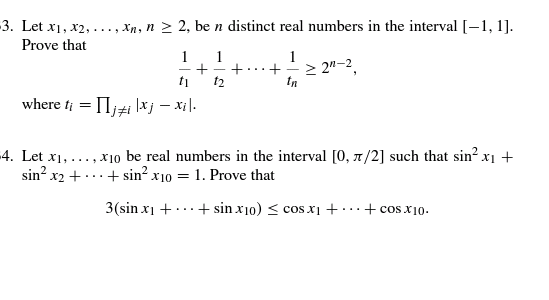# Doubt from trignometry@Hari_Shankar sir , @Jagdish_Singh sir i m not getting any idea for 1 st second i did but i need your approch please see it

For (4)

We have Given \displaystyle \sum^{10}_{i=1}\sin^2(x_{i})=1

Using

\displaystyle \cos(x_{i})=\sqrt{1-\sin^2(x_{i})}=\sqrt{\sin^2(x_{2})+\sin^2(x_{3})+\cdots+\sin^2(x_{10})}

By power mean Inequality

\displaystyle \sqrt{\frac{\sin^2(x_{2})+\sin^2(x_{3})+\cdots+\sin^2(x_{10})}{9}}\geq \bigg(\frac{\sin(x_{2})+\sin(x_{3})+\cdots+\sin(x_{10})}{9}\bigg).

\Longrightarrow \displaystyle \cos(x_{i})=\sqrt{\sin^2(x_{2})+\sin^2(x_{3})+\cdots+\sin^2(x_{10})}=\frac{\sin(x_{2})+\sin(x_{3})+\cdots+\sin(x_{10})}{3}.

Now do the same cyclic way, We have

\displaystyle \Rightarrow \color{Brown}\sum^{10}_{i=1}\cos(x_{i})\geq \frac{9}{3}\sum^{10}_{i=1}\sin(x_{i})=3\sum^{10}_{i=1}\sin(x_{i}).

3 Likes

thanks a lot sir your approch just astonish my brain sir thanks again see above to for this i also thought of power mean...

@Hari_Shankar sir sorry for again tagging you please if you got any idea please share your approch thanks sir.....